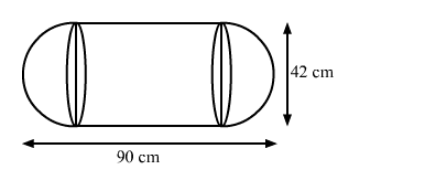# A toy is in the form of a cylinder with hemispherical ends.`
Question:

A toy is in the form of a cylinder with hemispherical ends. If the whole length of the toy is 90 cm and its diameter is 42 cm, then find the cost of painting the toy at the rate of 70 paise per sq cm.

Solution:We have,

the total height of the toy $=90 \mathrm{~cm}$ and

the radius of the toy, $r=\frac{42}{2}=21 \mathrm{~cm}$

Also, the height of the cylinder, $h=90-42=48 \mathrm{~cm}$

Now, the total surface area of the toy $=$ CSA of cylinder $+2 \times$ CSA of a hemisphere

$=2 \pi r h+2 \times 2 \pi r^{2}$

$=2 \pi r(\mathrm{~h}+2 r)$

$=2 \times \frac{22}{7} \times 21 \times(48+2 \times 21)$

$=44 \times 3 \times(48+42)$

$=44 \times 3 \times 90$

$=11880 \mathrm{~cm}^{2}$

So, the cost of painting the toy $=\frac{11880 \times 70}{100}=$ Rs 83,16